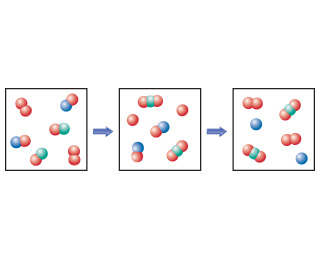# Problem: The following diagram represents an imaginary two-step mechanism. Let the red spheres represent element A, the green ones element B, and the blue ones element C.Identify the intermediate.

###### FREE Expert Solution

We are being asked to determine the intermediate in the diagram representing an imaginary two-step mechanism.

Recall: An intermediate is a compound that forms from the reactants and further reacts to form the products. We do not see it in the overall reaction as a main product.

The following are the given reaction mechanisms from the diagram

2 A2 + 2 AB + 2 AC  2 A2B + 2 A + 2 AC  2 A2B + 2 C + 2 A2

93% (72 ratings)###### Problem Details

The following diagram represents an imaginary two-step mechanism. Let the red spheres represent element A, the green ones element B, and the blue ones element C.Identify the intermediate.